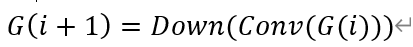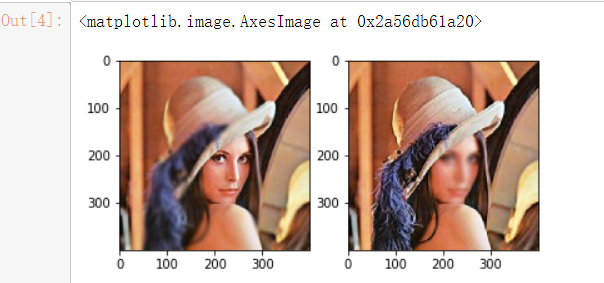# 拉普拉斯金字塔与高斯金字塔

2020/09/15 22:49

## 图像金字塔import cv2,numpy as np
import matplotlib.pyplot as plt

plt.subplot(121)
plt.imshow(莱娜1)
plt.subplot(122)
plt.imshow(莱娜2)#插值修改大小为2的倍数

temp=莱娜1.copy()
for index in range(层数):
dst = cv2.pyrDown(temp)
def 创建拉普拉斯金字塔(path,level):
img=cv2.cvtColor(img,cv2.COLOR_RGB2BGR)
img= cv2.resize(img,(512,512),interpolation=cv2.INTER_CUBIC)
temp = img.copy()#拷贝图像
高斯金字塔 = []
高斯金字塔.append(img)
for i in range(1,level):
下采样 = cv2.pyrDown(temp)
高斯金字塔.append(下采样)
temp = 下采样.copy()
拉普拉斯金字塔 = [高斯金字塔[level-1]]
for i in range(level-1,0,-1):
上采样 = cv2.pyrUp(高斯金字塔[i])
第L层的高斯金字塔 = cv2.subtract(高斯金字塔[i-1],上采样)
拉普拉斯金字塔.append(第L层的高斯金字塔)
print('高斯金字塔层数:',len(高斯金字塔),'拉普拉斯金字塔:',len(拉普拉斯金字塔))
return 拉普拉斯金字塔
def 拉普拉斯卷积(原图,卷积核大小):
卷积核=np.ones([卷积核大小,卷积核大小])*1/(卷积核大小*卷积核大小)
卷积图 = np.zeros([原图.shape-(卷积核大小-卷积核大小//2),原图.shape-(卷积核大小-卷积核大小//2)])
print(原图.shape)
原图行数,原图列数=原图.shape
for y in range(卷积核大小//2,原图行数-(卷积核大小-卷积核大小//2)):
for x in range(卷积核大小//2,原图列数-(卷积核大小-卷积核大小//2)):
半径=卷积核大小//2
拉普拉斯核 = np.array(([-1,-1,-1],[-1,8,-1],[-1,-1,-1]))
窗口=np.sum(原图[x-半径:x+半径+1,y-半径:y+半径+1]*拉普拉斯核)
卷积图[x, y] = 窗口
return 卷积图

plt.subplot(121)
plt.imshow(莱娜1卷积图,'gray')
plt.subplot(122)
plt.imshow(莱娜2卷积图,'gray')


0
0 收藏

### 作者的其它热门文章0 评论
0 收藏
0